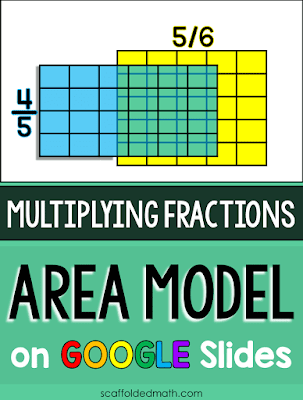## Pages

### Teaching Area Model Fraction Multiplication Digitally with GOOGLEIn this post I want to share with you a digital way to teach fraction multiplication with the area model, even from afar! The interactive GOOGLE Slides resource allows you and your students to move and overlap fractions to show fraction multiplication with the area model-- online and in color! And the editable GOOGLE Forms activity lets students revise their work when working through fraction multiplication problems.

Before we get into multiplying fractions with Google activities, I want to take a second to talk about why and how fraction multiplication works with a short video. In the video I show fraction multiplication with visual models and link it to multiplication of whole numbers.

### Fraction Multiplication with GOOGLE Slides

I made a YouTube video showing how you can use this new interactive fraction multiplication Google Slide to show multiplying fractions conceptually with the area model:

The blue and yellow fractions overlap on the grid as green, giving a nice visual representation of the product. You'll be able to easily show fraction multiplication as part of your distance lesson and also send the Slide to students to practice on their own. If there are 4 blue fractions and 5 yellow fractions, how many products are possible to show?

### Fraction Multiplication with GOOGLE FormsI also made a GOOGLE Forms fraction multiplication activity where students can practice what they have learned about multiplying fractions with the area model. There are 20 questions, each with 2 images. The first (above) asks students to 1) Multiply the fractions and 2) Simplify their answer.Each problem's second image asks students if they feel they got their answer correct. There is a multi-choice question to answer here: "Looking at the [color] picture, does the boxed region represent your answer?" Students can click "Yes, it does!" or "No, I will go back and try again". The Form allows students to go back and revise answers after being helped by the color picture.

I hope these two resources make it easier to teach, review and practice fraction multiplication with the area model.

When school is back in session, or to give students working at home (decorating the house with math sounds nice!), I also have this multiplying fractions math pennant where students color to show the overlapping areas. This one is nice because it doubles as classroom décor.Fraction review digital escape room

I've been working on building a collection of digital math escape rooms, like this fraction review digital escape room. Teachers are leaving lots of positive feedback about how much their students are enjoying these digital activities! Each digital math escape room is built in GOOGLE Slides with no outside links, making them super easy to assign to students.

If you are unsure how to use Google resources with students, here are some blog posts to help: